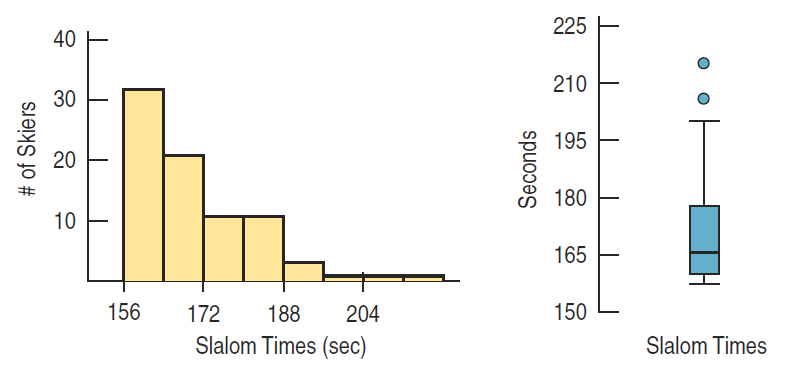×
Get Full Access to Stats: Data And Models - 4 Edition - Chapter 4 - Problem 18e
Get Full Access to Stats: Data And Models - 4 Edition - Chapter 4 - Problem 18e

×

# Slalom times 2010 The Men’s Giant Slalom skiing eventISBN: 9780321986498 70

## Solution for problem 18E Chapter 4

Stats: Data and Models | 4th Edition

• Textbook Solutions
• 2901 Step-by-step solutions solved by professors and subject experts
• Get 24/7 help from StudySoup virtual teaching assistantsStats: Data and Models | 4th Edition

4 5 1 420 Reviews
29
4
Problem 18E

Problem 18E

Slalom times 2010 The Men’s Giant Slalom skiing event consists of two runs whose times are added together for a final score. Two displays of the giant slalom times in the 2010 Winter Olympics are shown below.a) What features of the distribution can you see in both the histogram and the boxplot?

b) What summary statistic would you choose to summarize the center of this distribution? Why?

c) What summary statistic would you choose to summarize the spread of this distribution? Why?

Step-by-Step Solution:

Problem 18E

Slalom times 2010 The Men’s Giant Slalom skiing event consists of two runs whose times are added together for a final score. Two displays of the giant slalom times in the 2010 Winter Olympics are shown below.a) What features of the distribution can you see in both the histogram and the boxplot?

b) What summary statistic would you choose to summarize the center of this distribution? Why?

c) What summary statistic would you choose to summarize the spread of this distribution? Why?

Step by step solution

Step 1 of 3

(a)

One of the variables measured in the 2010 The Men’s Giant Slalom skiing event is Slalom times in second. And the other variable is the number of Skiers. We have given a histogram and 5-number summary of the event.

The distribution is unimodal and skewed to the right. The Median Slalom time is about 165 seconds. The minimum Slalom times is 156 and maximum of 215. We also see two possible outliers with Slalom times of 215 and 206 . Almost half of the number of skiers the Slalom time is between 160 and 178.

Step 2 of 3

Step 3 of 3

##### ISBN: 9780321986498

Unlock Textbook Solution